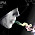Foundation, Concrete and Earthquake Engineering

What is the Significance of Modulus Elasticity of Concrete?

The definition and graphical interpretation of modulus of elasticity is common to all. We are concerned here, what is the significance of this in concrete engineering. The significance of it for materials that are homogeneous, defines inter-atomic forces of bond.

For such materials the microstructure is not important i.e. independent of microstructure properties. The concrete is not the same material as it is a heterogeneous material which has multiple phases in microstructure.

The typical range of modulus of elasticity of concrete under compression lies between (14X103– 40X103) Mpa. In FPS unit this lies between (2X106 – 6X106) psi.

In structural design, the practical significance of elastic limit depicts the fact which represents the allowable maximum stress of that material before undergoing permanent deformation.

The theoretical concept is the slope up to straight line portion of Stress-strain (σ-ε) plot i.e. within the region of elastic deformation.

Thus the higher modulus of elasticity of a material represents a stiffer one. This is a measure for resistance against elastic deformation. So the engineer should know this value of concrete as it have influence on rigidity of design member.

Dear reader we will discuss modulus of elasticity of concrete in respect of microstructural point of view and how microstructure influence modulus of elasticity.

1 comment:

1.Thanks for sharing. I hope it will be helpful for too many people that are searching for this topic. yunxuange.net